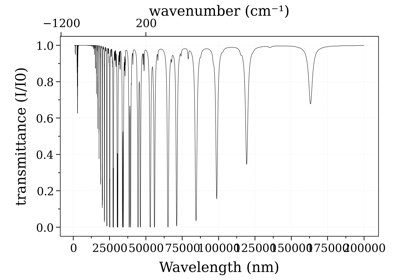## Module contents¶

Line-of-sight (multislabs) module for 1-D radiative transfer.

MergeSlabs(*slabs, **kwargs) [source]

Combines several slabs into one. Useful to calculate multi-gas slabs. Linear absorption coefficient is calculated as the sum of all linear absorption coefficients, and the RTE is recalculated to get the total radiance. You can also simply use:

s1//s2


Merged spectrum 1+2 is calculated with Eqn (4.3) of the [RADIS-2018] article, generalized to N slabs :

\begin{align}\begin{aligned}j_{\lambda, 1+2} = j_{\lambda, 1} + j_{\lambda, 2}\\k_{\lambda, 1+2} = k_{\lambda, 1} + k_{\lambda, 2}\end{aligned}\end{align}

where

$j_{\lambda}, k_{\lambda}$

are the emission coefficient and absorption coefficient of the two slabs 1 and 2. Emission and absorption coefficients are calculated if not given in the initial slabs (if possible).

Parameters

slabs (list of Spectra, each representing a slab) – path_length must be given in Spectrum conditions, and equal for all spectra.

line-of-sight:

slabs
        \====
light         ->     )===  observer
[n]        /====

Other Parameters
• kwargs input

• resample ('never', 'intersect', 'full') – what to do when spectra have different wavespaces:

• If 'never', raises an error

• If 'intersect', uses the intersection of all ranges, and resample spectra on the most resolved wavespace.

• If 'full', uses the overlap of all ranges, resample spectra on the most resolved wavespace, and fill missing data with 0 emission and 0 absorption

Default 'never'

• out ('transparent', 'nan', 'error') – what to do if resampling is out of bounds:

• 'transparent': fills with transparent medium.

• 'nan': fills with nan.

• 'error': raises an error.

Default 'nan'

• optically_thin (boolean) – if True, merge slabs in optically thin mode. Default False

• verbose (boolean) – if True, print messages and warnings. Default False

• modify_inputs (False) – if True, slabs are modified directly when they are resampled. This avoids making a copy so is slightly faster. Default False.

Returns

Spectrum – observed at the output. Conditions and units are transported too, unless there is a mismatch then conditions are dropped (and units mismatch raises an error because it doesnt make sense)

Return type

object representing total emission and total transmittance as

Examples

Merge two spectra calculated with different species (physically correct only if broadening coefficients dont change much):

from radis import calc_spectrum, MergeSlabs
s1 = calc_spectrum(...)
s2 = calc_spectrum(...)
s3 = MergeSlabs(s1, s2)


The last line is equivalent to:

s3 = s1//s2


Load a spectrum precalculated on several partial spectral ranges, for a same molecule (i.e, partial spectra are optically thin on the rest of the spectral range):

from radis import load_spec, MergeSlabs
spectra = []
for f in ['spec1.spec', 'spec2.spec', ...]:
s = MergeSlabs(*spectra, resample='full', out='transparent')
s.update()   # Generate missing spectral quantities
s.plot()Calculate a large spectrum by part

Calculate a large spectrum by part
SerialSlabs(*slabs: Spectrum, **kwargs: dict) [source]

Adds several slabs along the line-of-sight. If adding two slabs only, you can also use:

s1>s2


Serial spectrum 1>2 is calculated with Eqn (4.2) of the [RADIS-2018] article, generalized to N slabs :

\begin{align}\begin{aligned}I_{\lambda, 1>2} = I_{\lambda, 1} \tau_{\lambda, 2} + I_{\lambda, 2}\\\tau_{\lambda, 1+2} = \tau_{\lambda, 1} \cdot \tau_{\lambda, 2}\end{aligned}\end{align}

where

$I_{\lambda}, \tau_{\lambda}$

are the radiance and transmittance of the two slabs 1 and 2. Radiance and transmittance are calculated if not given in the initial slabs (if possible).

Parameters
• slabs (list of Spectra, each representing a slab) –

line-of-sight:

slabs              ............... [n]
:       :                    :         \====
light        *   ->  *        ->          *    ->    )===  observer
/====

• resample_wavespace ('never', 'intersect', 'full') – what to do when spectra have different wavespaces:

• If 'never', raises an error

• If 'intersect', uses the intersection of all ranges, and resample spectra on the most resolved wavespace.

• If 'full’, uses the overlap of all ranges, resample spectra on the most resolved wavespace, and fill missing data with 0 emission and 0 absorption

Default 'never'

• out ('transparent', 'nan', 'error') – what to do if resampling is out of bounds:

• 'transparent': fills with transparent medium.

• 'nan': fills with nan.

• 'error': raises an error.

Default 'nan'

Other Parameters
• verbose (bool) – if True, more blabla. Default False

• modify_inputs (False) – if True, slabs wavelengths/wavenumbers are modified directly when they are resampled. This avoids making a copy so it is slightly faster. Default False.

Note

for large number of slabs (in radiative transfer calculations) you surely want to use this option !

Returns

Spectrum – observed at the output (slab[n+1]). Conditions and units are transported too, unless there is a mismatch then conditions are dropped (and units mismatch raises an error because it doesnt make sense)

Return type

object representing total emission and total transmittance as

Examples

Add s1 and s2 along the line of sight: s1 –> s2:

s1 = calc_spectrum(...)
s2 = calc_spectrum(...)
s3 = SerialSlabs(s1, s2)


The last line is equivalent to:

s3 = s1>s2